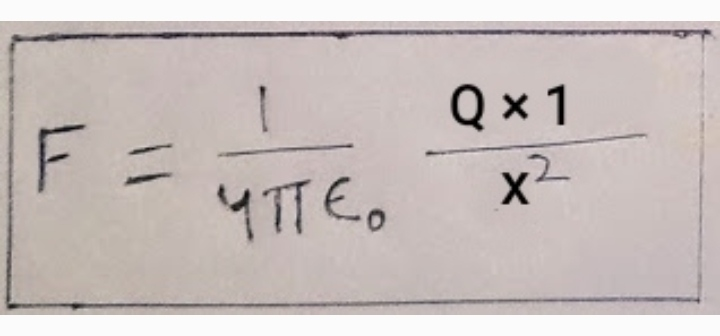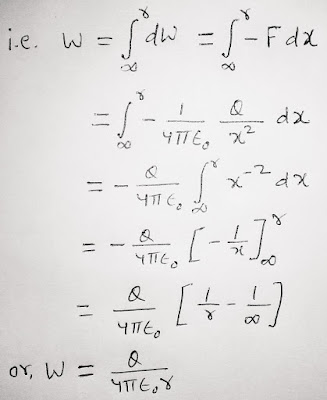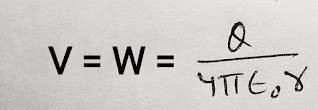# ( by integration method ) :

Consider a point charge + Q at any point and an electric field associated with this charge. we have to calculate the Electric Potential at any point P in this field due to  this point charge +Q.

Let us consider an unit positive charge ( +1 C ) at point A as shown in fig.

At point A  where OA = x , the electrostatic force on charge of 1 C is…….1

Now, if we want to move this unit charge towards the charge Q to a point P then we have to apply a force towards Q such that this force just cancel out the electrostatic force of repulsion due to charge Q  i.e. equal to F.

By applying this force, the unit charge moved to a point B from A ,as the distance travelled by unit charge is dx, then the work done to move unit charge with distance dx  is

( NOTE : ( - ) sign came in the expression because the direction of displacement dx is opposite to the direction of electrostatic force F, hence the angle between them is 180°. )

Now, as we know that the electric potential at point P is the amount of work done in bringing a unit positive charge from infinity to point P.

Hence, the total work done in moving + 1 C charge from ∞ to point P can be obtained by integrating equation (2) from ∞ to P. ( How to use integration method ? )And according to definition, this work for a unit charge is the Electric Potential at P due to charge Q, hence....……( 3 )

This is the final expression for the Electric potential at any point at a distance r due to a charge Q.